# Different Types of Pressure Gauge

Sharing is Caring :)-

Pressure is defined as the normal force exerted by a fluid per unit area of the surface. This pressure can be measured with various techniques using different types of devices. In case of fluid mechanics, we use some specific pressure gauges for measurement of pressure. Selection of pressure gauges depends upon the location and type of fluid flow. We will further discuss various types of pressure devices which we use nowadays for measuring fluid pressure in pipes, open channel and also discuss the techniques of measuring atmospheric pressure and pressure gauges which helps in measurement of atmospheric pressure.

Pressure is measured by the principle of manometry and the various devices used to measure pressure are known as manometers and the branch of science which deals with pressure measurement is known as manometry.

# Types of Pressure Gauge:

The different types of pressure measuring devices mainly classified in two categories_

1. Manometers
2.  Mechanical Gauges

### A.) MANOMETERS:

Manometers are the simple type of pressure measuring devices which works on the principle of balancing the column of liquid with the same or some another column of liquid. In this using one column we measure the pressure irrespective of the second column. It has further two types.

1. Simple Maometers
2. Differential Manometers

SIMPLE MANOMETER:

Simple manometer are very simple in construction they have a single glass tube with one end open to atmosphere and second end is attached to gauge points whose pressure is to be measured. By measuring the rise of liquid in simple manometer we can easily calculate the pressure. Simple manometer has three common types.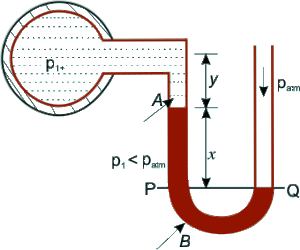• Piezometer
• U-tube manometer
• Single column manometer

PIEZOMETER:

Piezometer is the simple type of manometer which is used to measure the medium pressure range of liquids. A piezometer is a simple glass tube which is open at the both ends. One end of the piezometer is connected to the pipe line, vessel which containing liquid where pressure is needed to be measure and the other end kept open to the atmosphere. The glass tube must be attached vertically upwards and the liquid can freely rise in it without overflowing. The pressure of the liquid easily measured by height of the liquid rise in the tube using scale attached to it. Piezometer is used to measure gauge pressure only because surface of liquid is in contact with atmospheric pressure. The drawback of piezometer is, it can’t be used to measure very high pressure and gas pressure because one end is open.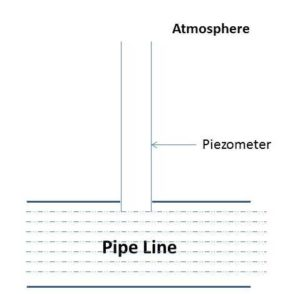U-TUBE MANOMETER:

Piezometer has some limitations these limitations are overcome by U-tube manometer like piezometer cannot measure high pressure for that piezometer require very long tubes which cannot provide easily and gas pressure is not measure by piezometers. By using U-tube manometer we can do both things. A U-tube manometer consists of a glass tube which is bent in ‘U’ shape due to this shape we call it as U-shape manometer. The one end of this manometer is open to atmosphere and other end is connected to the gauge points i.e. the point for which the pressure has to be measure. The tube of the U-tube manometer always filled with some other fluid which has higher specific gravity as compare to that fluid of which pressure is to be measure. This fluid is known as manometric fluid. Generally these fluids are mercury, salt solution, oil, carbon disulphide etc. We use these manometry fluids as per our requirements. Mercury is the very common manometric fluid because of its low vapour pressure. We can use more than one fluid in the manometers. These fluids should have different value of specific gravity, otherwise they get mixed. For measuring low pressure we use fluids have low value of specific gravity and for measuring high pressures we prefer those fluids which has high value of specific gravities like mercury. We can measure the pressure by rise of liquid or by measuring the height of liquid in the column.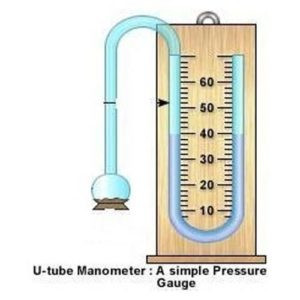SINGLE COLUMN MANOMETER:

Single column manometer is the modified form of U-tube manometer. In U-tube manometer we have to notice two readings i.e. rising of fluid in one limb and fall of fluid into second limb this difficulty is overcome in case of single column manometer. Here a reservoir of large cross sectional area having 100 times area than tube is attached with one side of the limb. When pressure varies the change in the level of liquid in the reservoir is very small but the variation of pressure can be easily noted in the attached limb with the help of attached scale. The rise or fall of the liquid level gives indication about the change in pressure. The narrow limb can be attached vertical or inclined as per requirements. Inclined type limb used to measure very small change in the pressure.

DIFFERENTIAL MANOMETER:

It is used to measure the difference of pressure between two points in a pipeline or in two separate pipes. Differential manometers consist of two bend glass tubes whose ends are connected to gauge points between the pressure differencehas to be measured. Following are the different types of differential manometers which are commonly used.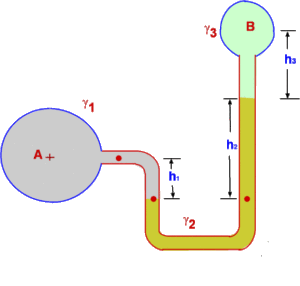• Two Piezometer Manometer
• Inverted U-tube Manometer
• U-Tube Differential Manometer

TWO PIEZOMETER MANOMETER:

As per name this consists of two piezometer which are mounted at the two different gauge points where pressure difference has to be measured. It has some limitations as piezometer. The pressure difference between two points can be easily measured by the difference in the levels of liquid raised in the two tubes.

INVERTED U-TUBE MANOMETER:

Inverted U-tube manometers are used when pipelines are under ground and in these manometers the density of manometric fluid is less than the density of flowing fluid. As name suggests it consists of a glass tube bent in U-shape which is held inverted. In most of the cases air is used as manometric fluid in them because of its low density. The points of this manometer are connected to gauge points whose pressure difference has to be measured. The difference in height of the liquids of both the limbs gives the pressure difference. An air clock is attached at the top of the inverted U-tube which helps in rising of the liquid to suitable height in both the limbs by driving out a position of the compressed air. Inverted U-tube manometers are used to measure small pressure difference in the liquids.

U-TUBE DIFFERENTIAL MANOMETER:

As name suggests it consists of a glass tube which is bend in U-shape. The two different ends of this tube are connected to the two gauge points between which the pressure difference is to be measured. At the middle of this tube or we can say at the lower part of the U-tube manometric fluid is filled having high specific gravity as compare to the liquids whose pressure difference has to be measured. By measuring the heights in two limbs we can easily measure the pressure difference by using U-tube differential manometer.

### B.) MECHANICAL GAUGES:

Mechanical gauges are used to measure very high pressure but having less accuracy. All the mechanical gauges consist of an elastic element which gets deformed on application of pressure. This deformation is further magnified by gear and pinion arrangement to a pointer scale and the pressure is measured. Following are the most commonly used pressure gauges.

• Bourdon Tube pressure gauge
• Diaphragm pressure gauge
• Bellows pressure gauge

BOURDON TUBE PRESSURE GAUGE:

Bourdon type is the most commonly type of pressure gauge. It is made up of steel or bronze tube. This tube is known as pressure responsive element. One end of this tube is closed and the other end is connected with pressure inlet. Initially the cross section of this tube is elliptical, but when the gauge is connected to the gauge point fluid under pressure enters into the tube, due to this the shape of the tube change from elliptical to circular. The closed end of the tube is free to move. The small movement of the free end of the tube is transmitted by using some linkage to the pointer which moves in clockwise direction. This pointer indicates the actual pressure of the fluid by using dial indicator. The dial gauge can be read zero value when the pressure is equal to atmospheric pressure. When pointer goes in anticlockwise it means that it shows negative gauge pressure or vacuum pressure.DIAPHRAGM PRESSURE GAUGE:

This device is used to measure generally low pressure intensity as compared to Bourdon tube. Here elastic steel corrugated diaphragm is the pressure responsive element. Its working is little bit similar to Bourdon tube the pressure is indicated by using needle which is moved with the help of pinion arrangement with the diaphragm.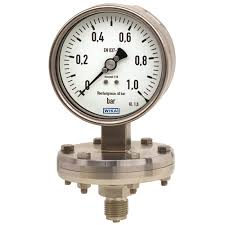BELLOWS PRESSURE GAUGE:

In this device the pressure responsive elements is made up of thin metal tube with deep circumferential corrugations when pressure changes the elastic elements expands or contracts. A pointer is attached with it which indicates the reading on circular dial. When pressure increases it expands and when pressure decreases the elastic elements in bellows pressure gauges are contracts.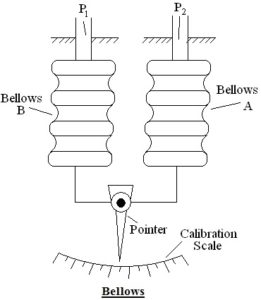Sharing is Caring :)-

### 3 thoughts on “Different Types of Pressure Gauge”

•June 1, 2019 at 6:18 pm

tank you for gud inform

•May 27, 2021 at 7:07 am

Hi sir
Pressure gauges only three types or more?
Bellow pressure gauges
Bourdon pressure gauges
DIAPHRAGM PRESSURE
•